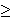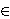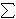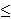# Nim GamesI enjoy going to schools to give talks. Generally, I try to focus these talks around mathematics that’s not generally taught in classrooms to try to connect to some of the inquisitive nature of the students. One of my favorite ways of doing this is through combinatorial games. These combinatorial games are generally two player sequential games (i.e. players alternate taking moves) where both players know all the information about the game before any moves are made. This is called a game of complete information. In addition, these games are deterministic, in that unlike a game of poker or dice there is no random element introduced into the game.

One of the most common ways of introducing students to combinatorial games is through the game of Nim (which is also called the Subtraction game). I’ve written a script here to help introduce this game. In the game of Nim, there are initially a number (p) of rocks in a pile. There is also an array of possible legal moves that each player can choose from on each turn. Players alternate removing a legal amount of stones from the pile until some player is unable to make a move, at which point the opposing player (the player who made the last move) is declared the winner.

So example a game of (1-2-3)-Nim could go as follows. Suppose initially there are 23 stones.

 Stones Player Removed 23 1 3 20 2 1 19 1 2 17 2 2 15 1 1 14 2 3 11 1 2 9 2 1 8 1 3 5 2 2 3 1 1 0 2 1

In the above example, since player 2 removes the last stone, player 1 is unable to move so player 2 is declared the winner. Each move that a player makes is either removing 1, 2 or 3 stones as we initially stated in the rules of the game.

Because Nim is a game of perfect information, we know a lot about the game before any moves are made. In fact, we can determine who should win the game if it is played perfectly just by knowing the set of available moves and the number of stones in the pile. We can do this by considering a game with 0 stones and determining who would win this game (player 2), and increasing the number of stones in the pile one by one and at each new cell, determining who would be the winner. In this method, we can say that we are in a winning position if there is a feasible move that would put the opposing player into a losing position. Consider the following table for the (1-2-3)-Nim game:

 Stones 0 1 2 3 4 5 6 7 8 9 10 11 12 13 14 15 16 17 18 19 20 21 22 23 Winner 2 1 1 1 2 1 1 1 2 1 1 1 2 1 1 1 2 1 1 1 2 1 1 1

We can analyze this table as follows. With 0 stones, there are no moves that any player can make, but since player 1 goes first, they cannot make a move and lose the game. When there is 1, 2, or 3 stones, then player 1 can remove all the stones in the pile and in all cases player 2 will be looking at a situation where there are no stones to remove. When there are 4 stones, no matter how many stones player 1 removes, player 2 will be able to remove the remaining stones to ensure that player 1 is looking at a situation with no stones. We can repeat this process with any number of stones and we arrive at a table similar to the one listed above.

I have a script at my Nim games page where the set of possible moves and the number of stones in the pile are generated randomly and users get to play against a computer. Check it out and let me know what you think.

# Assembly Line Scheduling

I wanted to take a minute to help some users become more familiar with Dynamic Programming, so I decided to write a script on the Assembly Line Scheduling Problem.

To introduce the problem I want to tell you a story about a friend of mine. Keisha recently started a clothing company that uses two assembly lines to produce articles of clothing. She has separated the the process of manufacturing an item of clothing into n steps, so each assembly line is separated into n different stations, with each station performing a specific task (So for example station three’s job may be to add a right sleeve to shirts). The task of a specific station is independent of which line the station occurs on (so if station three’s job is to add a right sleeve to shirts, this will be true in both assembly line 1 and assembly line 2). Lets denote the jth station (with j = 1, 2, …, n) on line i (where i is 1 or 2) by Si, j. Although they’re doing the same jobs the time it takes the employee at station S1, j may be different from the time it takes the employee at station S2, j. We will denote the time required at station Si, j by ai, j. For each line, there is also an amount of time required for the article of clothing to enter assembly line i, ei; and an amount of time required for the article of clothing to exit assembly line i, xi.

One of the reasons that assembly lines are very productive is that stations on the same assembly line are generally in close proximity to one another, resulting in a very low cost of transferring an item from one station to the next on the same assembly line. When we have multiple lines in place, as Keisha has, there is a (possibly beneficial) cost of transferring an item from one line to another. Lets denote this cost by ti, j which represents the cost of transferring a partially completed item of clothing from line i after having gone through station Si, j (again, i is 1 or 2 and j = 1, 2, …, n).

The problem that Keisha would like solved is to determine which station to choose between lines 1 and 2 in order to minimize the total time it takes to produce an article of clothing.

Consider the following example:Our goal is to get the clothing through the 3 states to produce a final product. What if we initially had the product take the route through station S2, 1 instead of station S1, 1? Lets assume that we make the decisions to send the article of clothing to stations S2, 2 and S2, 3 afterwards. This would result in a solution whose total cost is 3 + 8 + 4 + 6 + 3 = 24. Is this solution optimal (aka is this solution the minimum total time through the factory)? Lets consider what would happen if we had chosen station S1, 1 instead of S2, 1. The entry cost for line 1 is 1, the time required at station S1, 1 is 5 and the transfer time to go to assembly line 2 is 1. So the cost of this new solution is 1 + 5 + 1 + 4 + 6 + 3 = 20, which gives a cheaper solution.

This is called the principle of optimality (optimal substructure property) which states that in order for an overall solution to be optimal, the solution must also give the optimal solutions to every subproblem of the original problem. This problem of solving all subproblems may seem like a daunting task at first, but lets consider the example above again.

Initially, we have a new product and there are two options – either line one or line two. We will need these values in the future, so lets keep track of both choices in the form of a table.

 Station 1 cost0 e1 + a1, 1 cost1 e2 + a2, 1

After this initial step, the question becomes given the current path to station j-1, which assembly line can best serve station j? This cam be computed for each j > 1 by
cost1(j) = min{cost1(j-1) + a1, j, cost2(j-1) + t2, j-1 + a1, j}
cost2(j) = min{cost2(j-1) + a2, j, cost1(j-1) + t1, j-1 + a2, j}

As you can see, the calculation of costi(j) relies on the computation of costi(j-1). By calculating these values from station 1 to to station n, we are able to simply look up the values in the table instead of having to recalculate these values.

These give optimal solutions to each of the subproblems. We repeat this same step for all stages j = 2, …, n then we arrive at the final step were we finish the job. Lets define total_cost to indicate the cost of the optimal solution.
total_cost = min{cost1(n) + x1, cost2(n) + x2}

We’d like to see which value minimizes total_cost. Then we can trace back to find the values that minimized cost1 or cost2 at each step depending on which assembly line was chosen. The following algorithm does just this, and stores the assembly line chosen at each state in the variable line.

For the above example, the table would be calculated as follows:

 Station 1 Station 2 Station 3 Total Cost cost1 6 13 18 21 cost2 11 11 17 20

We can reconstruct the optimal path through assembly lines by seeing that we finish by going through station S2, 3.
We arrive at station S2, 3 by going through the assembly line station S2, 2.
We arrive at station S2, 2 by going through the assembly line station S1, 1.

This is precisely the path that is highlighted in the image above.

The algorithm to construct these paths and compute the total_cost for such problems is given below.

Algorithm FastestWay(a, t, e, x, m)
cost1 [<-] e1 + a1, 1
cost2 [<-] e2 + a2, 1
for (j [<-] 2 to n) if (cost1(j-1) + a1, j [<=] cost2(j-1) + t2, j-1 + a1, j
cost1(j) [<-] cost1(j-1) + a1, j
line1(j) [<-] 1 else cost1(j) [<-] cost2(j-1) + t2, j-1 + a1, j
line1(j) [<-] 2if (cost2(j-1) + a2, j [<=] cost1(j-1) + t1, j-1 + a2, j
cost2(j) [<-] cost2(j-1) + a2, j
line2(j) [<-] 1 else cost2(j) [<-] cost1(j-1) + t1, j-1 + a2, j
line2(j) [<-] 2if (cost1(n) + x1 [<=] cost2(n) + x2)
total_cost = cost1(n) + x1
final_line = 1
else
total_cost = cost2(n) + x2
final_line = 2

Note: I used Introduction to Algorithms by Thomas H. Cormen, Charles E. Leiserson, Ronald L. Rivest, and Clifford Stein to help with this post.

# Approximating the Set Cover ProblemI just finished my weekly task of shopping for groceries. This can be a somewhat daunting task because I generally have a list of things that I’ll need which cannot all be purchased at a single location. What often happens is that I find that many of the items on my list are ONLY offered at certain stores – generic brands of certain items for example. My goal then changes from minimizing the total amount of money spent to minimizing the number of stores that I must visit to purchase all of my items.

To formulate this as a mathematical problem, suppose that I have a grocery list of items I would like to buy, represented by the lists item1, item2, …, itemn, where n represents the number of items I have on this list. Suppose also that there are stores Store1, Store2, …, Storem (each one distinct) that offer some combination of items I have on my list. What I would like to do is minimize the number of stores I have to visit to purchase these items.

The problem I just described is famous because it is one that many people face on a regular basis. In a more general form, it is so famous that it has a name for it, called the Set Cover Problem (or the Minimum Set Cover Problem). In the general form of this problem, we replace the grocery list with a set of items called our universe. The lists of items offered at each store are the collections of subsets of the universe. In the problem, as in the example above, we would like to select enough subsets from this collection that we are able to obtain every element in our universe. We would like to do this with as low a number of sets as possible.

In my previous post, I described the 21 problems that Karp proved were NP-Complete. Set Cover was one of those problems, showing that this is a hard problem to solve. What I will do is introduce three ways to reach a near-optimal solution relatively quickly.

Greedy Method

One of the first approaches one may take to solve this problem is to repeatedly select the subset that contains the most new items. That’s how the greedy approach to set cover operates. The method knows to terminate when all elements belong to one of the selected sets. In the shopping example above, this would be accomplished by visiting the store that had the most items on my list and purchasing those items at this store. Once this is done, the items that have been purchased can be crossed off my list and we can visit the store with the most items on my remaining list, stopping when the list is empty.

Linear Programming Relaxation

Instead of stating the set cover problem with words, there is a way of describing the situation with mathematical inequalities. For instance, suppose that the soap I like to purchase is only available at stores Store1, Store4 and Store9. Then I could introduce a variable xi for each store i and the requirement that I purchase this soap can be restated as :

x1 + x4 + x91

Because we can either purchase some items or not purchase these items, each variable xi is 0 or 1 (called a binary variable). We can introduce similar constraints for each element in our universe (or on our grocery list). These inequalities (called constraints) have the form:

for each element eU,i | eSi xi1

Our goal of minimizing the number of sets chosen (stores visited) can be stated by the objective function:
minimize1in xi

So the mathematical formulation for this problem can be stated as

minimize1in xi
Subject to
for each element eU,i | eSi xi1
for each set i, xi{0, 1}.

Formulations of this type, where variables are restricted to a finite set (in this case the x variables being either 0 or 1) are called integer programs. Unfortunately, there is no easy way to solve these formulations either. However, there is a related problem which can be solved quickly.

Instead of restricting the x variables to the values of 0 or 1, we could allow them to take on any value within this range, i.e. 0xi1 for each set Si. Doing this converts the problem from an integer programming problem into a linear programming problem (called the LP-Relaxation), which can be solved quickly. The issue with this method though is that the solution obtained by an LP-Relaxation is not guaranteed to be an integer. In this case, how do we interpret the values xi?

Randomized Rounding Method

One approach to dealing with a non-integer solution to the LP-Relaxation is to treat the xi values as probabilities. We can say that xi is the probability that we select set i. This works because each value of xi is in the range of 0 to 1, which is necessary for a probability. We need to repeatedly select sets with their associated probabilities until all elements in our universe are covered. Selecting our sets based on this procedure is the randomized rounding approach.

Deterministic Rounding Method

A second approach to dealing with a non-integer solution to the LP-Relaxation is to base our solution on the most occurring element. If we let f be this frequency (i.e.the number of sets that the most occurring element occurs in), then we can define a solution by selecting set i if the LP=Relaxation solution gives the variable xi a value of at least (1/f).

None of these three approaches is guaranteed to give an optimal solution to an instance of this problem. I will not go into it in this post, but these can all be shown to be within some guaranteed range of the optimal solution, thus making them approximation algorithms.

Hope you enjoy.

# Knapsack ProblemsTo help understand this problem, I want you to think about a common situation in many people’s lives. You have a road trip coming up today and you’ve overslept and are at risk of missing your flight. And to top matters off, you were planning to pack this morning but now do not have the time. You quickly get up and begin to get ready. You grab the first bag you see and quickly try to make decisions on which items to take. In your head you’re trying to perform calculations on things you’ll need for the trip versus things that you can purchase when you get there; things that you need to be able to have a good time versus things you can do without. And to top matters off, you don’t have time to look for your ideal luggage to pack these things. So you have the additional constraint that the items you pick must all fit into this first bag you found this morning.

The situation I described above is a common problem. Even if we ignore the part about the flight, and just concentrate on the problem of trying to put the most valuable set of items in our bag, where each item has its own value and its own size limitations, this is a problem that comes up quite often. The problem is known (in the math, computer science and operations research communities) as the knapsack problem. It is known to be difficult to solve (it is said to be NP-Hard and belongs to a set of problems that are thought to be the most difficult problems within its class). Because of this difficulty, this problem has been well studied.

What I provide in my script are two approaches to solving this problem. The first is a greedy approach, which selects a set of items by iteratively choosing the item with the highest remaining value to size ratio. This approach solves very fast, but can be shown to give sub-optimal solutions.

The second approach is a dynamic programming approach. This algorithm will solves the problem by ordering the items 0, 1, …, n and understanding that in order to have the optimal solution on the first i items, the optimal solution must have been first selected on the fist i-1 items. This algorithm will optimally solve the problem, but it requires the computation of many sub-problems which causes it to run slowly.

Update (4/2/2013): I enjoy this problem so much that I decided to implement two additional approaches to the problem: Linear Programming and Backtracking.

The Linear Programming approach to this problem comes from the understanding that the knapsack problem (as well as any other NP-Complete problem) can be formulated as an Integer Program (this is a mathematical formulation where we seek to maximize a linear objective function subject to a set of linear inequality constraints with the condition that the variables take on integer values). In the instance of the knapsack problem we would introduce a variable xi for each item i; the objective function would be to maximize the total value of items selected. This can be expressed as a linear objective function by taking the sum of the products of the values of each item vi and the variable xi; the only constraint would be the constraint saying that all items fit into the knapsack. This can be expressed as a linear inequality constraint by taking the sum of the products of the weights of each item wi and the variable xi. This sum can be at most the total size of the knapsack. In the integer programming formulation, we either select an item or we do not. This is represented in our formulation by allowing the variable xi = 1 if the item is selected, 0 otherwise.

The LP relaxation of an integer program can be found by dropping the requirements that the variables be integer and replacing them with linear equations. So in the case of the knapsack problem, instead of allowing the variables to only take on values of 0 and 1, we would allow the variables to take on any value in the range of 0 and 1, i.e 0 <= xi <= 1 for each item i. We can then solve this LP to optimality to get a feasible solution to the knapsack problem.The second knapsack approach I implemented today is through backtracking. Similar to the Dynamic Programming approach to this problem, the backtracking approach will find an optimal solution to the problem, but these solutions generally take a long time to compute and are considered computationally inefficient. The algorithm I implemented here first orders the item by their index, then considers the following sub-problems for each item i "What is the best solution I can obtain with this initial solution?". To answer this question, the algorithm begins with an initial solution (initially, the empty set) and a set of unchecked items (initially, all items) and recursively calls itself on sub-problems with an additional item as a part of the initial solution and with this item removed from the unchecked items.So check out my knapsack problem page. I think its a good way to be introduced to the problem itself, as well as some of the techniques that are used in the fields of mathematics, computer science, engineering and operations research.

Other Blogs covering this topic:
Journey to the Four Point Oh